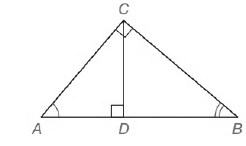Chapter 5.CT, Problem 6CT### Elementary Geometry for College St...

6th Edition
Daniel C. Alexander + 1 other
ISBN: 9781285195698

#### Solutions

Chapter
Section### Elementary Geometry for College St...

6th Edition
Daniel C. Alexander + 1 other
ISBN: 9781285195698
Textbook Problem
1 views

# In right triangle A B C ,   C D - is the altitude from C to hypotenuse A B - . Name three triangles that are similar to each other. ___________To determine

To find:

The triangles that is similar to each other.

Explanation

Given,

A right triangle ABC, CD¯ is the altitude from C to hypotenuse AB¯.

Here, the altitude cd¯ is drawn to the hypotenuse of a right triangle ABC that separates the right triangle into two right triangles as ADC,CDB

Therefore, we have the smaller triangles as follows,

Consider the ABC,ACD

Here, AA

BC

CD

AAA Theorem:

If the three angles of one triangle are congruent to the three angles of a second triangle then the triangles are similar.

Therefore by the above theorem

ABC~ACD

Consider the ABC,CBD

### Still sussing out bartleby?

Check out a sample textbook solution.

See a sample solution

#### The Solution to Your Study Problems

Bartleby provides explanations to thousands of textbook problems written by our experts, many with advanced degrees!

Get Started

#### Convert the expressions in Exercises 8596 radical form. 34/5

Finite Mathematics and Applied Calculus (MindTap Course List)

#### Find f in terms of f and g. h(x) = f(g(sin 4x))

Single Variable Calculus: Early Transcendentals, Volume I

#### In Exercises 1520, simplify the expression. 17. 16x5yz481xyz54; x 0, y 0, z 0

Applied Calculus for the Managerial, Life, and Social Sciences: A Brief Approach

#### In Problems 1-6 simplify each fraction. 6.

Mathematical Applications for the Management, Life, and Social Sciences

#### Use a Table of Integrals to evaluate .

Study Guide for Stewart's Single Variable Calculus: Early Transcendentals, 8th